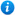20% OFF MEMBERSHIP THIS WEEKEND - Enter promo code MEM20 for 20% off

# Introducing Multiplying 2-Digits by 1-Digit - Year 3

Introducing Multiplying 2-Digits by 1-Digit - Year 3

Year 3 maths programme of study - Number - Number (multipliction and division)

• recall and use multiplication and division facts for the 3, 4 and 8 multiplication tables
• write and calculate mathematical statements for multiplication and division using the multiplication tables that they know
• solve problems, including missing number problems, involving multiplication and division
In this maths teaching resource, pupils are introduced to multiplying a 2-digit number by a 1-digit number (no exchange). Children use their knowledge of repeated addition to represent a 2-digit number multiplied by a 1-digit number using pictorial representations. These activities cover the year 3 curriculum objectives listed above and support the White Rose small steps guidance for year 3 - Spring - Block 1 - Multiplication and Division. The resource consists of 1 lesson, including a PowerPoint presentation and differentiated worksheets. The worksheets contain varied fluency, reasoning and problem solving to reinforce and consolidate the children's learning. Content includes:
• Varied fluency and reasoning and problem solving interactive class activities
• 3 differentiated varied fluency and reasoning and problem solving worksheets with answers

'Introducing Multiplying 2-Digits by 1-Digit - Year 3' is completely editable giving teachers the freedom to adapt the resource to suit their individual teaching needs.

Click on the images from the PowerPoint presentation to view the resource in more detail.

Our Price : £2.99 / 3 Credits## Get this resource as part of a bundle and save up to 52%## Year 3 Multiplication and Division Bundle 2

£9.99

By continuing to use the site, you agree to the use of cookies. You can change this and find out more by following this link